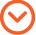11/29/2016 By Andrew PetryszakIn an earlier post on inferential statistics, I wrote about defining a population and constructing a random sample. In the example described, KCIC was tasked with confirming that invoice information contained in a spreadsheet had hardcopy invoices to back up the numbers. The population was defined as all the individual dollars in the spreadsheet report. I explained how to construct a random sample from this population. In this post, I will describe some factors to consider in choosing a sample size, as well as the resulting potential effects on the analyses.

Determining Sample Size
To determine a sample size, we must consider both the confidence level and the margin of error. These two statistical terms are interdependent. The confidence level states the percent of certainty, or probability, that the population’s expected value falls within a given range, centered around the sample’s mean value. The margin of error defines how wide that range is. The confidence interval is the range bounded by the average sample value minus the margin of error (lower bound) and the average sample value plus the margin of error (upper bound).

In statistics, 95 percent and 99 percent confidence levels are the most commonly used because of their higher levels of certainty. Using a 99 percent confidence level would mean that 99 out of 100 times, the expected value for the population would fall within the confidence interval. For example, if in our sample we found invoice evidence 85 percent of the time, and our margin of error was 1 percent for a 99 percent confidence level, then we could say that 99 out of 100 times, we expect that the number of dollars with invoice evidence will be between 84 and 86 percent of the population’s total.

Solving for the Desired Variable
It would seem logical that we would want the highest possible confidence level, with the lowest possible margin of error, in order to get the most accurate and reliable assessment of the population’s true value. The trick is that for a given level of confidence, the margin of error is inversely dependent upon the sample size. The larger the sample, the lower the margin of error and vice versa. Computing the minimum sample size for a given confidence level and given margin of error, or computing the margin of error for a given confidence level and given sample size, depends a little more on the situation:In our example, because we do not know the population’s standard deviation, we would use a t-table, or t-distribution, to find the “t-value.” Then we can use one of the above equations to solve for either the margin of error or the minimum sample size by choosing a value for the other variable, calculating the sample’s standard deviation, and looking up the t-value for the chosen level of confidence.

In this instance, as in real life, the sample size is often constrained by resources. Using this equation, we can calculate our margin of error for different sample sizes and choose one that is acceptable for the given circumstances.

Once we determine the appropriate confidence level, margin of error, and sample size, we can begin to infer information about the population of invoice dollars. In my next post, I will discuss calculating the confidence interval and drawing statistical conclusions. It is these conclusions that give us valuable information to be used in expert reports and other analyses to help our clients gain valuable insights, while looking at only a fraction of a population of data points. Learn more about KCIC’s Consulting services here.